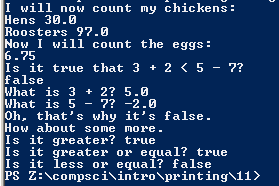Program #11

## Code

```
/// Name: Afaf Nabeeha
/// Period: 7
/// Program Name: Numbers and Math
/// File Name: Numbers.java
/// Date Finished: 9/17/2015 public class Numbers
{
public static void main( String[] args )
{
System.out.println( "I will now count my chickens:" );

// The next line totals the number of hens//
System.out.println( "Hens " + ( 25.0 + 30.0 / 6.0 ) );
// The next line totals the number of roosters//
System.out.println( "Roosters " + ( 100.0 - 25.0 * 3.0 % 4.0 ) );

System.out.println( "Now I will count the eggs:" );
// The next line uses addition, subtraction, division and percentages to total the total number of eggs//
System.out.println( 3.0 + 2.0 + 1.0 - 5.0 + 4.0 % 2.0 - 1.0 / 4.0 + 6.0 );
// The next line is asking a question that will be answered in line 18
System.out.println( "Is it true that 3 + 2 < 5 - 7?" );
// This line determines if the previous question is true or false//
System.out.println( 3.0 + 2.0 < 5.0 - 7.0 );
// Lines 20 and 21 are asking for a computation and also provide an answer//
System.out.println( "What is 3 + 2? " + ( 3.0 + 2.0) );
System.out.println( "What is 5 - 7? " + ( 5.0 - 7.0 ) );

System.out.println( "Oh, that's why it's false." );

System.out.println( "How about some more." );
// The last few lines ask a question and get answered in the same line by using a "+"//
System.out.println( "Is it greater? " + ( 5.0 > -2.0 ) );
System.out.println( "Is it greater or equal? " + ( 5.0 >= -2.0 ) );
System.out.println( "Is it less or equal? " + ( 5.0 <= -2.0 ) );
}
}
```

### Picture of the output